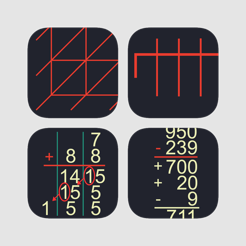## Screenshots

•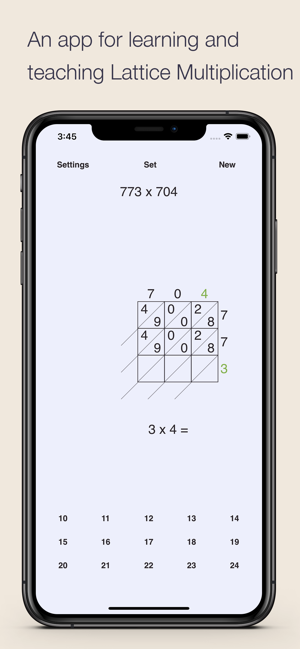•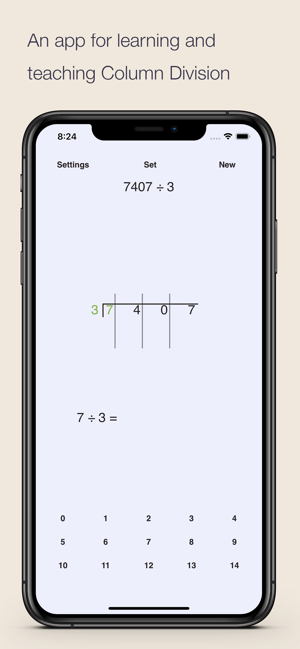•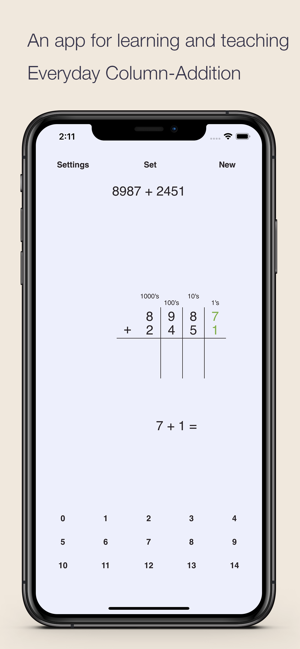•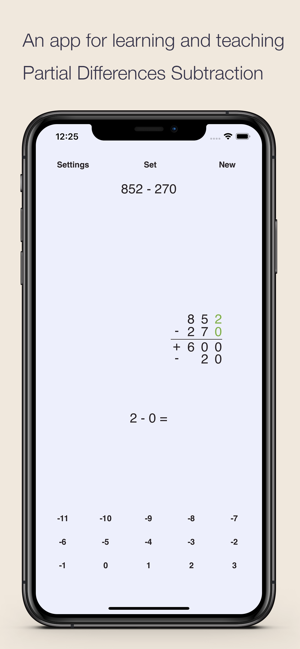•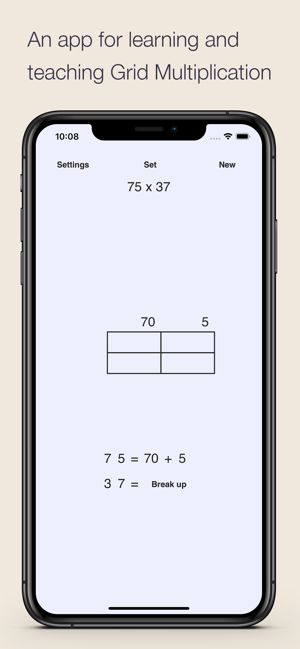•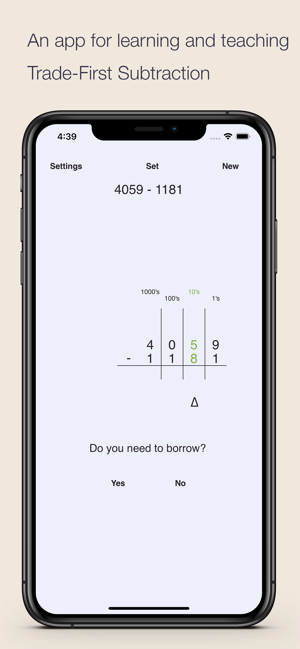•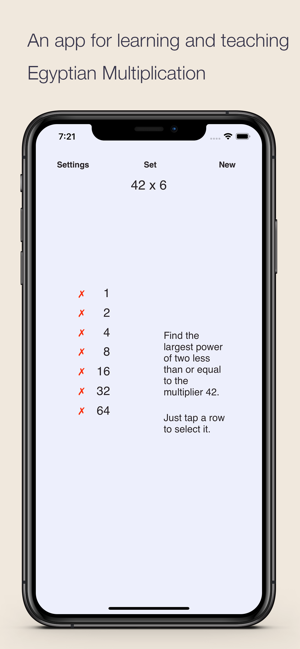•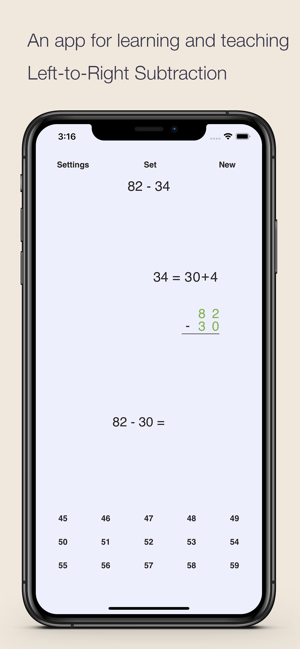•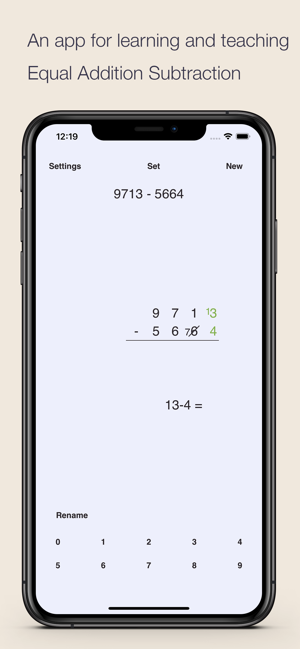•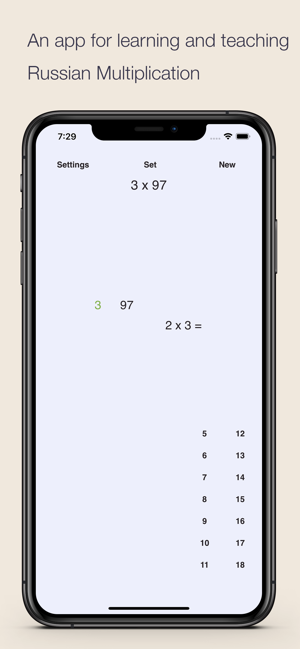## Description

This bundle has 10 iDevBooks math apps. Save 60% compared to buying the apps separately.

Lattice Multiplication

Lattice multiplication is algorithmically equivalent to long multiplication. A lattice (a grid) guides the calculation. All the multiplications are done first and then the additions. The method was introduced to Europe in 1202 in Fibonacci's Liber Abaci.

In this groundbreaking book Fibonacci presented many algorithms for working with Arabic numerals. Ancient Indians and Chinese originally invented some of the algorithms. Fibonacci presented both the current standard long multiplication and also an originally Indian method called lattice multiplication, which is faster and more compact for working with larger numbers.

Grid Multiplication

The grid multiplication method is a two-step process. In the example 39 x 24 the numbers are first broken up into 30 + 9 and 20 + 4, and written on a grid. Multiplying the row and column headers then solves each cell of the grid. Eventually the partial products 600, 180, 120, and 36 are added together.

Egyptian Multiplication

Ancient Egyptians were able to multiply any two numbers by using just the ability to multiply by 2, and to add.

Russian Multiplication

In this method is the the only multiplication facts we need to know are how to double and how to take half of a number.

Column Division

The column division method is a simple variation of the traditional long division method. Lines are drawn to separate the digits of the divisor. Each place-value column is solved from left to right.

The Everyday column-addition method was developed by a first grader. The method has two stages. In the first stage the digits are added one column at a time in each column. After the first stage some columns can have two-digit numbers. In the second stage the carries are done to obtain the result.

Partial Differences Subtraction

The partial differences subtraction method is a two-step process. In the example 936 - 580, each place value is first subtracted separately from left to right. The partial differences are added and 400 - 50 + 6 produces the answer 356.

Wired.com: “Esa Helttula’s apps are all fantastic for math learning and practice, and aren’t cluttered with mini-games, incentives, or annoying music.”

The trade-first subtraction method is similar to the traditional column subtraction method. The method has two stages. All of the "trading" or "borrowing" is done first. Then the subtractions are solved. This gives the method it's name, "trade-first."

The method is based upon the idea that adding the same amount to two numbers will not affect the difference between the two numbers. The process can be thought as:
- Work column by column from right to left
- When the digit of the first number is smaller than the corresponding digit of the second number, add ten to the digit of the first number and one to the digit of the second number in the next column to the left.

The equal addition subtraction method is also called the ‘borrow and repay’ method or equal additions method for subtraction. Today the method is used in Australia and Latin America. The equal addition used to be a very popular method for subtraction in US until the middle of the 20th century.

Left-to-Right Subtraction

The left-to-right subtraction method is a two-step process. In the example 936 - 581, the number to be subtracted is first written in an expanded form: 500+80+1. Each of the parts is then subtracted from the starting number. In our example 936-500=436, 436-80=356 and finally 356-1=355.

Privacy

The apps have no ads or in-app purchases and they do not transmit any data during the operation of the app. The apps also do not contain any links to other apps or the web.

Videos

Dozens of videos of these and other apps can be found at idevbooks.com.

## Ratings and Reviews

4.0 out of 5
1 Rating

1 Rating

bhima ,

### Left-to-right or Right-to-left

Simple demonstrations of various math procedures: Left-to-right subtraction, Trade-first subtraction, Equal addition subtraction, Partial differences, Column addition,
Russian multiplication, Lattice multiplication, Egyptian multiplication, Grid multiplication, Column division

In the settings you choose number of digits, animation speed, and other options.

## Information

Seller
Esa Helttula
Size
6.7 MB
Category
Education
Compatibility

Requires iOS 10.0 or later. Compatible with iPhone, iPad, and iPod touch.

Languages

English

Age Rating
4+
•